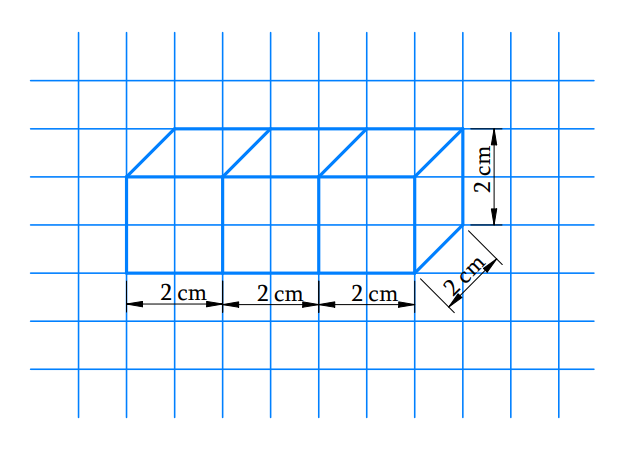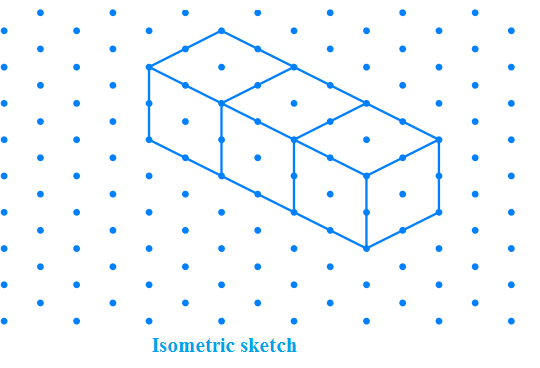# Ex.15.2 Q3 Visualising Solid Shapes - NCERT Maths Class 7

Go back to  'Ex.15.2'

## Question

Three cubes each with $$2$$ cm edge are placed side by side to form a cuboid. Sketch an oblique or isometric sketch of this cuboid.

Video Solution
Visualizing Solid Shapes
Ex 15.2 | Question 3

## Text Solution

What is known?

Three cubes each with $$2 \,\rm{cm}$$ edge are placed side by side to form a cuboid.

What is unknown?

Oblique or isometric sketch of this cuboid.

Reasoning:

Draw rough sketch of this cuboid and then draw oblique and isometric sketch.

Steps:

Length of three each cube edge $$=$$ $$2$$ cm

Placing three cubes side by side, then the dimension of cuboid $$= 6\rm{cm}×2 \rm{cm}×2 \rm{cm}$$Learn from the best math teachers and top your exams

• Live one on one classroom and doubt clearing
• Practice worksheets in and after class for conceptual clarity
• Personalized curriculum to keep up with school